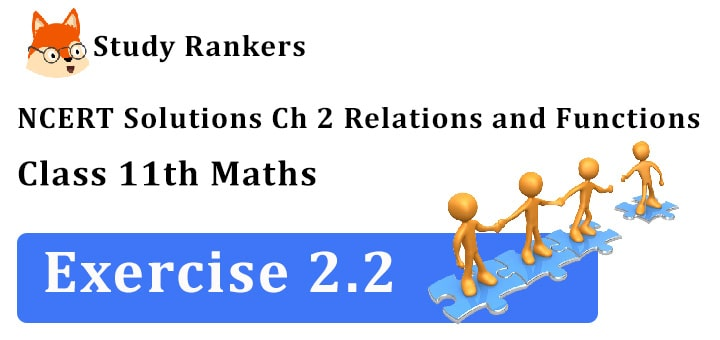## NCERT Solutions for Class 11 Maths Chapter 2 Relations and Functions Exercise 2.2

Chapter 2 Relations and Functions Exercise 2.2 Class 11 Maths NCERT Solutions will prove beneficial in understanding the basics of the chapter and prepare for examination in well manner. NCERT Solutions for Class 11 Maths provided here will solve your doubts if occur while solving any questions.1. Let A = {1, 2, 3,.... 14}. Define a relation R from A to A by R = {(x, y): 3x – y = 0 where x, y ∈ A}. Write down the domain, co-domain and range.

(i) By the definition of the relation, R = {(1, 3) (2, 6), (3, 9), (4, 12)}. The corresponding arrow diagram is:(ii) We can see that the set of first element i.e., the domain = {1, 2, 3, 4}.
Similarly, the set of second elements (images) i.e., the range = {3, 6, 9, 12} and the co-domain = {1, 2, 3,.... 14}.

2. Define a relation R on the set N of natural numbers by R = {(x, y): y = x + 5, x is a natural number less than 4, x, y  ∈ N}. Depict this relationship using (i) roster form (ii) an arrow diagram. Write down the domain and the range.

Here, R = {(x, y): y = x + 5, x is a natural number less than 4, x, y ∈ N}.
(i) In roster form, R = {(1, 6), (2, 7), (3, 8)}.
(ii) The arrow diagram is:Domain is the set of first elements i.e., {1, 2, 3}.
Range is the set of second elements i.e., {6, 7, 8}.

3. A = {1, 2, 3, 5} and B = {4, 6, 9}. Define a relation R from A to B by R = {(x, y): the difference between x and y is odd, x ∈ A, y ∈ B}. Write R is roster form.

Here A = {1, 2, 3, 5}and B = {4, 6, 9}
Relation R from A to B is given by
R = {(x, y): the difference between x and y is odd,
x ∈ A, y ∈ B}
∴ R = {(5, 4)}.

4. The figure shows a relationship between the sets P and Q. Write this relation (i) in set builder form, (ii) roster form. What is its domain and range?It is obvious that the relation R is “the difference between x and y is 2”.
In roster form, R = {(5, 3), (6, 4), (7, 5)}.
In set builder form, R = {(x, y): x – y = 2, x ∈ P, y ∈ Q}.
The domain of this relation is {5, 6, 7}.
The range of this relation is {3, 4, 5}.

5. Let A = {1, 2, 3, 4, 6}. Let R be the relations on A defined by {(a, b)}: a, b ∈ A, a divides b}.
(i) Write R in roster form
(ii) Find the domain of R
(iii) Find the range of R

(i) Here A = {1, 2, 3, 4, 6} and R is a relation on A defined by {(a, b): a ∈ A, b ∈ A, a divides Clearly, R = {(1, 1), (1, 2), (1, 3), (1, 4), (1, 6), (2, 2), (2, 4), (3, 3), (3, 6), (4, 4), (6, 6)}.
(ii) Domain = {1, 2, 3, 4, 6}.
(iii) Range = {1, 2, 3, 4, 6}.

6. Determine the domain and range of the relation R defined by R = {(x, x + 5): x ∈ {(0, 1, 2, 3, 4, 5)}.

Here,
R = {(x, x + 5): x ∈ (0, 1, 2, 3, 4, 5)}
∴ Domain = {0, 1, 2, 3, 4, 5}
[On putting x = 0, 1, 2, 3, 4, 5]
and Range = {5, 6, 7, 8, 9, 10}
[On putting x = 0, 1, 2, 3, 4, 5 in x + 5].

7. Write the relation R = {(x, x3): x is prime number less than 10} in roster form.

Here, R = {(x, x3): x is a prime number less than 10}
∴ R = {(2, 8), (3, 27), (5, 125), (7, 343)}.

8. Let A = {x, y, z} and B {1, 2}. Find the number of relations from A to B.

Given:
A = {x, y, z} and B = {1, 2}
A × B = {x, y, z} × {1, 2} = {(x, 1), (x, 2), (y, 1), (y, 2), (z, 1), (z, 2)}
Since n (A × B) = 6, the number of all relations in the set A × B = 26 i.e., 64.

9. Let R be the relation on Z defined by R = {(a, b): a ∈ Z, b ∈ Z, a – b is an even integer}. Find the domain and range of R.